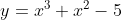## Inflection Point

For the curve represented by the equation below, what value of x does the only point of inflection occurs at?Hint
First, find the second derivative of $$f(x)$$ .
Hint 2
Set $$f''(x)=0$$ to solve for the inflection point.
An inflection point is a point on the curve/graph at which concavity changes, and occurs when $$f''(x)=0$$ .
Using the power rule for the first derivative and applying it twice, we’ll get the second derivative power rule:
$$\frac{d^2}{dx^2}[x^n]=\frac{d}{dx}\frac{d}{dx}[x^n]=\frac{d}{dx}[nx^{n-1}]=n\frac{d}{dx}[x^{n-1}]=n(n-1)(x^{n-2})$$$Thus, the second derivative is: $$f''(x)=3(3-1)x^{3-2}+2(2-1)x^{2-2}-0$$$
$$=3(2)x^{1}+2(1)x^{0}$$$$$f''(x)=6x+2(1)=6x+2$$$
Solving for $$x$$ when $$f''(x)=0$$ to get the x-component inflection point:
$$6x+2=0$$$$$x=-\frac{2}{6}=-\frac{1}{3}$$$
Since $$f''(x)=0$$ and $$f''(x)$$ changes signs at $$x=-1/3$$ , the inflection point is at $$x=-1/3$$ .
-1/3